##Projectivity fixing triangle vertices

Here we consider a special projectivity related to a special equilateral triangle. We identify the projective plane P2 with the projectification of the affine plane E of R3, defined through the equation x1+x2+x3=1. The line at infinity is described through the equation x1+x2+x3=0.
We consider the equilateral triangle T defined on E by the unit vectors e1, e2, e3 of R3. Its center is the point e=(1/3, 1/3, 1/3). The projectivity Fx fixing the vertices of the triangle and mapping e to x is defined through (non-zero multiples of) a matrix M. M is found by the conditions (i) M*ei = si*ei, for i=1,2,3 and (ii) M*e = s*x. These imply: si=s*xi, for i=1,2,3. Thus the matrix is a multiple of the diagonal matrix: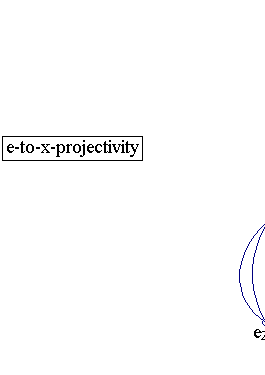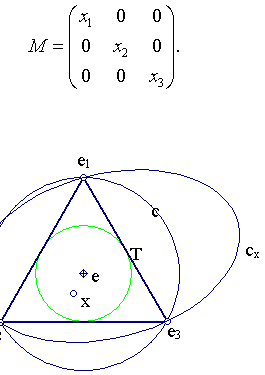Notice that x must not lie on the sides (prolongations) of the equilateral. This is the necessary condition for the definition of the projectivity Fx (each tripple out of the four {e1,e2,e3,x} must be non-collinear). The images c'=Fx(c) of the circumcircle c of T give all possible circumconics of T. For x different from e, this map is never an affinity. In fact it never fixes the line at infinity. This is seen from the equation (in variable y's) (i): x1*y1+x2*y2+x3*y3=0, which determines the pre-image of the line at infinity. The pre-image is the line I(x) defined as the intersection of the two planes (i) and (ii): y1+y2+y3=1.
The previous remark reveals also the various parts of the plane where the corresponding cx is an ellipse, hyperbola or parabola. In fact the parabola, touching the line at infinity, results when I(x) is tangent to the circumcircle c of the equilateral. This is equivalent with the tangency of plane (i) to the cone defined by c and the origin in R3. The corresponding normal x of these planes consequently traces on E a certain circle, which an easy calculation proves it to be the incircle of the equilateral. Thus for all x's inside the incircle we get ellipses, for all x's on the incircle we get parabolas and for all x's outside the incircle we get hyperbolas.
The above argument can be transferred, through conjugation via an affinity, to an arbitrary triangle T=ABC. In fact, consider the affinity F mapping the vertices of a fixed equilateral to the vertices of ABC and the center of the equilateral to the centroid G of ABC. Then the in/circum-circle of the equilateral are mapped by F to the corresponding inner/outer Steiner ellipses of ABC (see Steiner_Ellipse.html ). Then use projectivities of the form:
Gy = F*Fx*F-1, Fx as above for y=F(x),
Note that each of these circumconics can be directly defined through the projectivity mapping the vertices of the equilateral to the vertices of the triangle and the center of the equilateral to point y. to define all circumconics of ABC.
The above discussion shows that for all y inside the inner Steiner ellipse we get elliptic circumconics. For y on the inner Steiner ellipse we get parabolas circumscribed and for y outside the inner Steiner conic we get hyperbolas (see TriangleCircumconics.html ).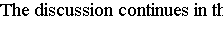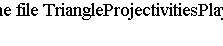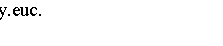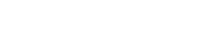CrossRatio.html
CrossRatio0.html
CrossRatioLines.html
GoodParametrization.html
Harmonic.html
Harmonic_Bundle.html
HomographicRelation.html
HomographicRelationExample.html
Pascal.html